# 1. blockCV introduction: how to create block cross-validation folds

## Introduction

The package `blockCV` offers a range of functions for generating train and test folds for k-fold and leave-one-out (LOO) cross-validation (CV). It allows for separation of data spatially and environmentally, with various options for block construction. Additionally, it includes a function for assessing the level of spatial autocorrelation in response or raster covariates, to aid in selecting an appropriate distance band for data separation. The `blockCV` package is suitable for the evaluation of a variety of spatial modelling applications, including classification of remote sensing imagery, soil mapping, and species distribution modelling (SDM). It also provides support for different SDM scenarios, including presence-absence and presence-background species data, rare and common species, and raster data for predictor variables.

Please cite `blockCV` by: Valavi R, Elith J, Lahoz-Monfort JJ, Guillera-Arroita G. blockCV: An R package for generating spatially or environmentally separated folds for k-fold cross-validation of species distribution models. Methods Ecol Evol. 2019; 10:225–232. doi: 10.1111/2041-210X.13107

## New updates of the version 3.0

The latest version `blockCV` (v3.0) features significant updates and changes. All function names have been revised to more general names, beginning with `cv_*`. Although the previous functions (version 2.x) will continue to work, they will be removed in future updates after being available for an extended period. It is highly recommended to update your code with the new functions provided below.

• Function names have been changed, with all functions now starting with `cv_`
• The CV blocking functions are now: `cv_spatial`, `cv_cluster`, `cv_buffer`, and `cv_nndm`
• Spatial blocks now support hexagonal (default), rectangular, and user-defined blocks
• A fast C++ implementation of Nearest Neighbour Distance Matching (NNDM) algorithm (Milà et al. 2022) is now added
• The NNDM algorithm can handle species presence-background data and other types of data
• The `cv_cluster` function generates blocks based on kmeans clustering. It now works on both environmental rasters and the spatial coordinates of sample points
• The `cv_spatial_autocor` function now calculates the spatial autocorrelation range for both the response (i.e. binary or continuous data) and a set of continuous raster covariates
• The new `cv_plot` function allows for visualization of folds from all blocking strategies using ggplot facets
• The `terra` package is now used for all raster processing and supports both `stars` and `raster` objects, as well as files on disk.
• The new `cv_similarity` provides measures on possible extrapolation to testing folds

## Installation

The `blockCV` is available in CRAN and the latest update can also be downloaded from GitHub. It is recommended to install the dependencies of the package. To install the package use:

``````# install stable version from CRAN
install.packages("blockCV", dependencies = TRUE)

# install latest update from GitHub
remotes::install_github("rvalavi/blockCV", dependencies = TRUE)``````
``````# loading the package
library(blockCV)``````
``## blockCV 3.1.3``

## Package data

The package contains the raw format of the following data:

• Raster covariates of Australia (`.tif`)
• Simulated species data (`.csv`)

These data are used to illustrate how the package is used. The raster data include several bioclimatic variables for Australia. The species data include presence-absence records (binary) of a simulated species.

To load the package raster data use:

``````library(sf) # working with spatial vector data
library(terra) # working with spatial raster data
library(tmap) # plotting maps

# the pipe operator |> is available for R version 4.1 or higher
rasters <- system.file("extdata/au/", package = "blockCV") |>
list.files(full.names = TRUE) |>
terra::rast()``````

The presence-absence species data include `243` presence points and `257` absence points.

``````# load species presence-absence data and convert to sf
points <- read.csv(system.file("extdata/", "species.csv", package = "blockCV"))
``````##            x        y occ
## 1  1313728.4 -2275453   0
## 2  1176795.0 -1916003   0
## 3 -1741599.3 -3927213   1
## 4  1099769.9 -4124055   1
## 5  1279495.1 -3901538   0
## 6   928603.1 -1342594   0``````

The appropriate format of species data for the `blockCV` package is simple features (from the `sf` package). The data is provide in GDA2020 / GA LCC coordinate reference system with `"EPSG:7845"` as defined by `crs = 7845`. We convert the `data.frame` to `sf` as follows:

``pa_data <- sf::st_as_sf(points, coords = c("x", "y"), crs = 7845)``

Let’s plot the species data using `tmap` package:

``````tm_shape(rasters[]) +
tm_raster(legend.show = FALSE, n = 30, palette = gray.colors(10)) +
tm_shape(pa_data) +
tm_dots(col = "occ", style = "cat", size = 0.1)``````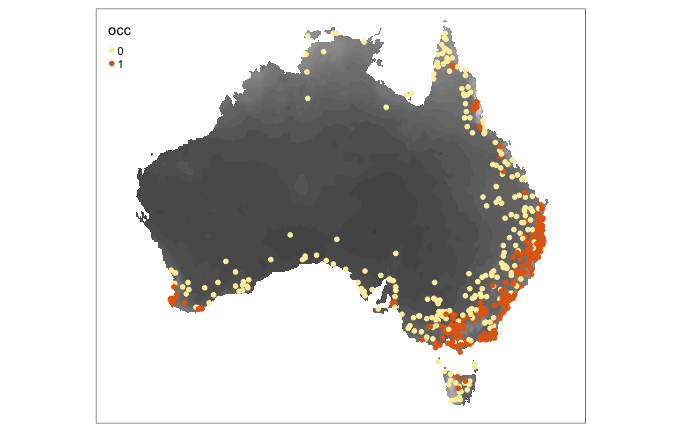## Block cross-validation strategies

The `blockCV` stores training and testing folds in three different formats. The common format for all three blocking strategies is a list of the indices of observations in each fold. For `cv_spatial` and `cv_cluster` (but not `cv_buffer` and `cv_nndm`), the folds are also stored in a matrix format suitable for the `biomod2` package and a vector of fold’s number for each observation. This is equal to the number of observation in spatial sample data (argument `x` in functions). These three formats are stored in the cv objects as `folds_list`, `biomod_table` and `folds_ids` respectively.

### Spatial blocks

The function `cv_spatial` creates spatial blocks/polygons then assigns blocks to the training and testing folds with random, checkerboard pattern or a systematic way (with the selection argument). When `selection = "random"`, the function tries to find evenly distributed records in training and testing folds. Spatial blocks can be defined either by `size` or number of rows and columns.

Consistent with other functions, the distance (`size`) should be in metres, regardless of the unit of the reference system of the input data. When the input map has geographic coordinate system (i.e. decimal degrees), the block size is calculated based on dividing `size` by 111325 (the standard distance of a degree in metres, on the Equator) to change metre to degree. In reality, this value varies by a factor of the cosine of the latitude. So, an alternative sensible value could be `cos(mean(sf::st_bbox(x)[c(2,4)]) * pi/180) * 111325`.

The `offset` argument can be used to shift the spatial position of the blocks in horizontal and vertical axes, respectively. This only works when the block have been built based on `size`, and the `extend` option allows user to enlarge the blocks ensuring all points fall inside the blocks (most effectve when `rows_cols` is used). The blocks argument allows users to define an external spatial polygon as blocking layer.

Here are some spatial block settings:

``````sb1 <- cv_spatial(x = pa_data,
column = "occ", # the response column (binary or multi-class)
k = 5, # number of folds
size = 350000, # size of the blocks in metres
selection = "random", # random blocks-to-fold
iteration = 50, # find evenly dispersed folds
biomod2 = TRUE) # also create folds for biomod2``````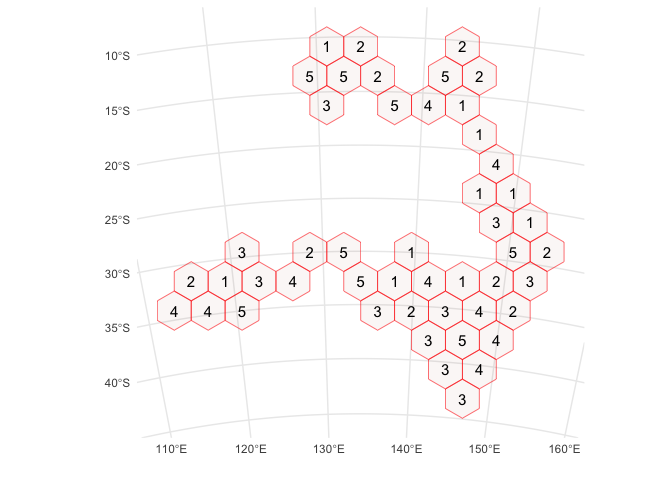The output object is an R S3 object and you can get its elements by a `\$`. Explore `sb1\$folds_ids`, `sb1\$folds_list`, and `sb1\$biomod_table` for the three types of generated folds from the `cv_spatial` object `sb1`. Use the one suitable for you modelling practice to evaluate your models. See the explanation of all other outputs/elements of the function in the help file of the function.

The same setting from previous code can be used to create square blocks by using `hexagon = FALSE`. You can optionally add a raster layer (using `r` argument) for to be used for creating blocks and be used in the background of the plot (raster can also be added later only for visualising blocks using `cv_plot`).

``````sb2 <- cv_spatial(x = pa_data,
column = "occ",
r = rasters, # optionally add a raster layer
k = 5,
size = 350000,
hexagon = FALSE, # use square blocks
selection = "random",
progress = FALSE, # turn off progress bar for vignette
iteration = 50,
biomod2 = TRUE)``````
``````##
##   train_0 train_1 test_0 test_1
## 1     211     157     46     86
## 2     192     213     65     30
## 3     216     201     41     42
## 4     210     202     47     41
## 5     199     199     58     44``````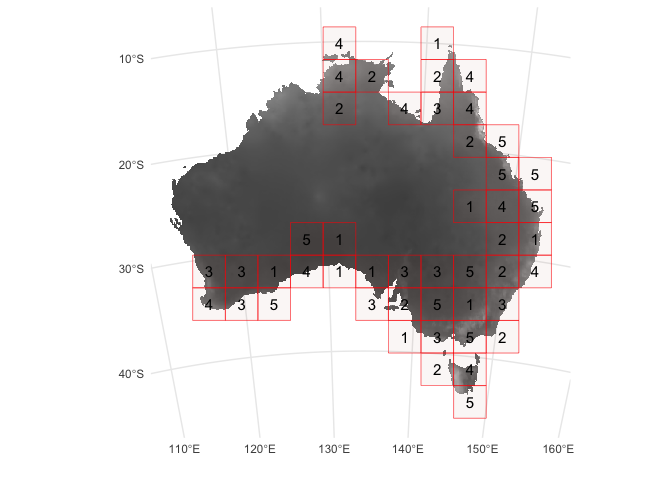The assignment of folds to each block can also be done in a systematic manner using `selection = "systematic"`, or a checkerboard pattern using `selection = "checkerboard"`. The blocks can also be created by number of rows and columns when no `size` is supplied by e.g. `rows_cols = c(12, 10)`.

``````# systematic fold assignment
# and also use row/column for creating blocks instead of size
sb3 <- cv_spatial(x = pa_data,
column = "occ",
rows_cols = c(12, 10),
hexagon = FALSE,
selection = "systematic")``````
``````##
##   train_0 train_1 test_0 test_1
## 1     227     203     30     40
## 2     204     232     53     11
## 3     215     125     42    118
## 4     194     220     63     23
## 5     188     192     69     51``````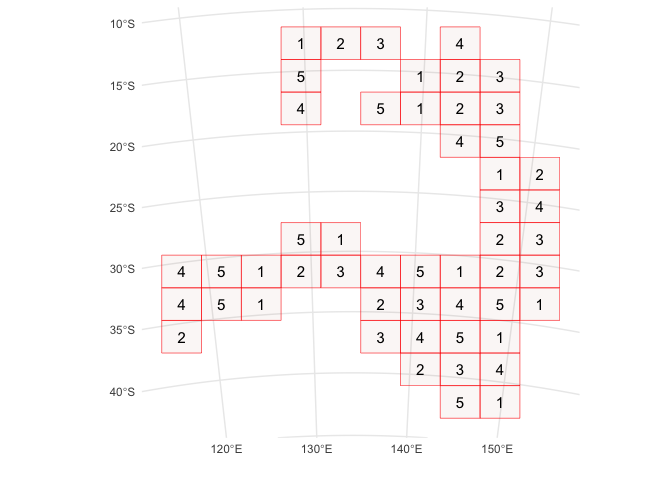The function’s output report reveals that setting the selection to ‘random’ results in a more even distribution of presence/absence instances between the train and test folds compared to ‘systematic’. This is because the random assignment process is repeated multiple times, controlled by the `iteration` parameter, to ensure that the folds are evenly distributed.

``````# checkerboard block to CV fold assignment
sb4 <- cv_spatial(x = pa_data,
column = "occ",
size = 350000,
hexagon = FALSE,
selection = "checkerboard")``````
``````##
##   train_0 train_1 test_0 test_1
## 1     125     143    132    100
## 2     132     100    125    143``````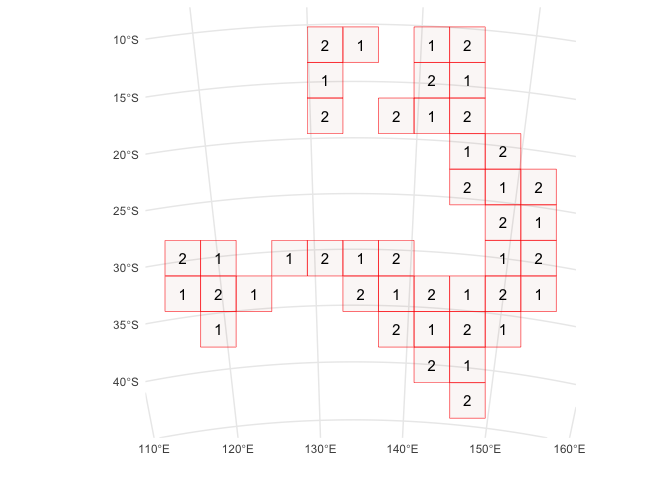Let’s visualise the checkerboard blocks with `tmap`:

``````tm_shape(sb4\$blocks) +
tm_fill(col = "folds", style = "cat")``````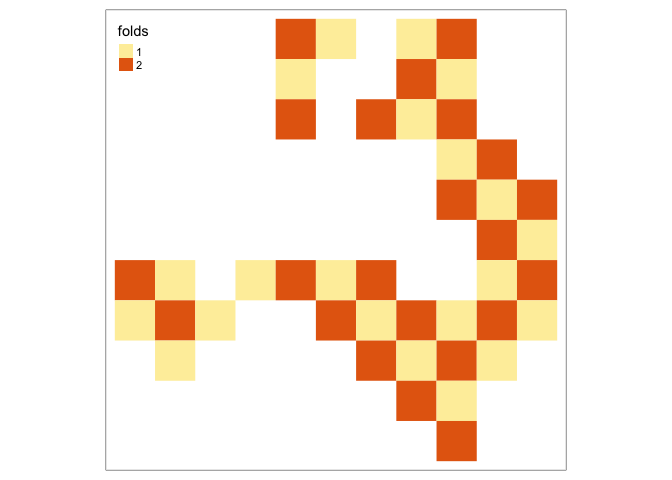### Spatial and environemntal clustering

The function `cv_cluster` uses clustering methods to specify sets of similar environmental conditions based on the input covariates. Species data corresponding to any of these groups or clusters are assigned to a fold. Alternatively, the clusters can be based on spatial coordinates of sample points (the `x` argument).

Using spatial coordinate values for clustering:

``````# spatial clustering
set.seed(6)
scv <- cv_cluster(x = pa_data,
column = "occ", # optional: counting number of train/test records
k = 5)``````
``````##   train_0 train_1 test_0 test_1
## 1     230     240     27      3
## 2     171     142     86    101
## 3     232     228     25     15
## 4     203     227     54     16
## 5     192     135     65    108``````

The clustering can be done in environmental space by supplying `r`. Notice, this could be an extreme case of cross-validation as the testing folds could possibly fall in novel environmental conditions than what the training points are (check `cv_similarity` for testing this). Note that the input raster layer should cover all the species points, otherwise an error will rise. The records with no raster value should be deleted prior to the analysis or a different raster be used.

``````# environmental clustering
set.seed(6)
ecv <- cv_cluster(x = pa_data,
column = "occ",
r = rasters,
k = 5,
scale = TRUE)``````
``````##   train_0 train_1 test_0 test_1
## 1     249     172      8     71
## 2     215     234     42      9
## 3     211     211     46     32
## 4     216     145     41     98
## 5     137     210    120     33``````

When `r` is supplied, all the input rasters are first centred and scaled to avoid one raster variable dominate the clusters using `scale = TRUE` option.

By default, the clustering will be done based only on the values of the predictors at the sample points. In this case, and the number of the folds will be the same as `k`. If `raster_cluster = TRUE`, the clustering is done in the raster space. In this approach, the clusters will be consistent throughout the region and across species (in the same region). However, this may result in cluster(s) that cover none of the species records especially when species data is not dispersed throughout the region (or environmental ranges) or the number of clusters (`k` or folds) is high.

### Buffering LOO (also known as Spatial LOO)

The function `cv_buffer` generates spatially separated training and testing folds by considering buffers of specified distance around each observation point. This approach is a form of leave-one-out (LOO) cross-validation. Each fold is generated by excluding nearby observations around each testing point within the specified distance (ideally the range of spatial autocorrelation). In this method the test set never directly abuts a training set.

Using buffering to create CV folds:

``````bloo <- cv_buffer(x = pa_data,
column = "occ",
size = 350000)``````

When using species presence-background data (or presence and pseudo-absence), you need to supply the `column` and set `presence_bg = TRUE`. In this case, only presence points (1s) are considered as target points. For more information read the details section in the help of the function (i.e. `help(cv_buffer)`).

For species presence-absence data and any other types of data (such as continuous, counts, and multi-class targets) keep `presence_bg = FALSE` (default). In this case, all sample points other than the target point within the buffer are excluded, and the training set comprises all points outside the buffer.

## Nearest Neighbour Distance Matching (NNDM) LOO

The `cv_nndm` is a fast implementation of the Nearest Neighbour Distance Matching (NNDM) algorithm (Milà et al., 2022) in C++. Similar to `cv_buffer`, this is a variation of leave-one-out (LOO) cross-validation. It tries to match the nearest neighbour distance distribution function between the test and training data to the nearest neighbour distance distribution function between the target prediction and training points (Milà et al., 2022).

``````nncv <- cv_nndm(x = pa_data,
column = "occ",
r = rasters,
size = 350000,
num_sample = 5000,
sampling = "regular",
min_train = 0.1,
plot = TRUE)``````
``````##     train_0         train_1          test_0          test_1
##  Min.   :210.0   Min.   :146.0   Min.   :0.000   Min.   :0.000
##  Mean   :244.4   Mean   :221.2   Mean   :0.514   Mean   :0.486
##  Max.   :257.0   Max.   :243.0   Max.   :1.000   Max.   :1.000``````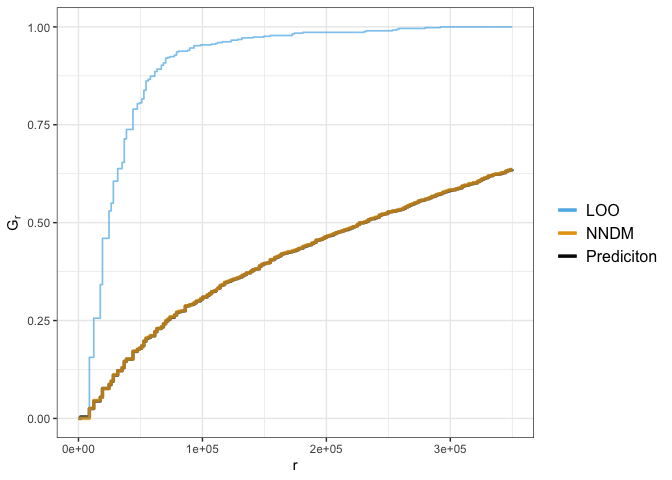## Visualising the folds

You can visualise the generate folds for all block cross-validation strategies. You can optionally add a raster layer as background map using `r` option. When `r` is supplied the plots might be slightly slower.

Let’s plot spatial clustering folds created in previous section (using `cv_cluster`):

``````cv_plot(cv = scv,
x = pa_data)``````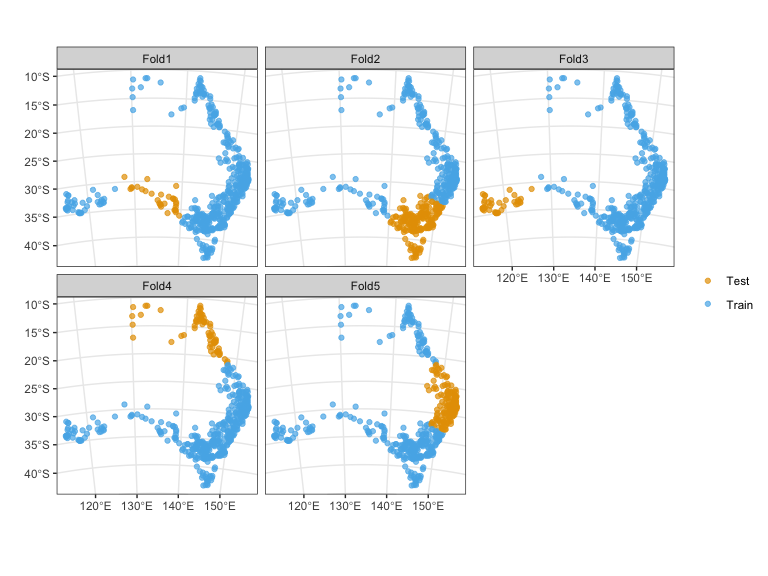When `cv_buffer` is used for plotting, only first 10 folds are shown. You can choose any set of CV folds for plotting. If `remove_na = FALSE` (default is `TRUE`), the `NA` in the legend shows the excluded points.

``````cv_plot(cv = bloo,
x = pa_data,
num_plots = c(1, 50, 100)) # only show folds 1, 50 and 100``````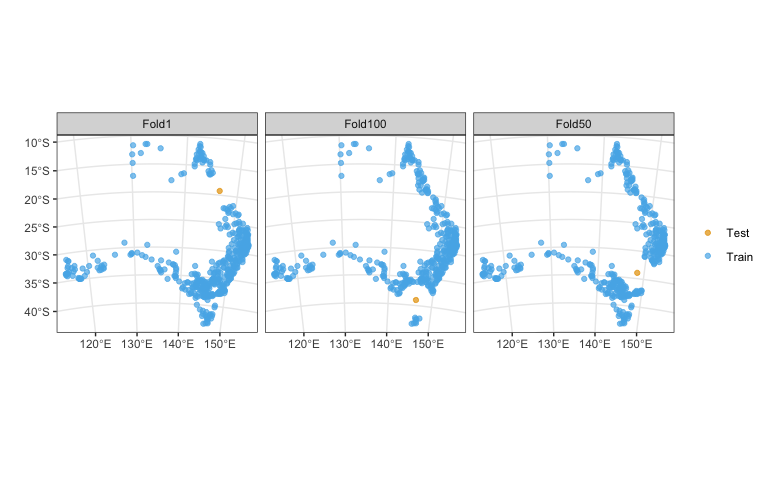If you do not supply `x` when plotting a `cv_spatial` object, only the spatial blocks are plotted.

``````cv_plot(cv = sb1,
r = rasters,
raster_colors = terrain.colors(10, alpha = 0.5),
label_size = 4) ``````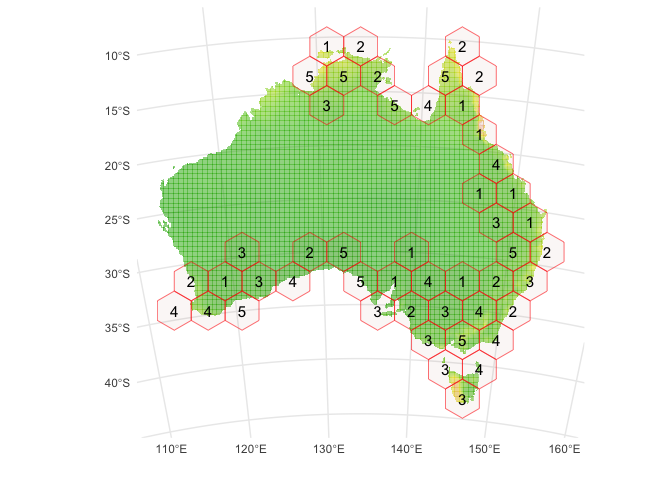## Check similarity

The `cv_similarity` function can check for environmental similarity between the training and testing folds and thus possible extrapolation in the testing folds. It computes multivariate environmental similarity surface (MESS) as described in Elith et al. (2010). MESS represents how similar a point in a testing fold is to a training fold (as a reference set of points), with respect to a set of predictor variables in `r`. The negative values are the sites where at least one variable has a value that is outside the range of environments over the reference set, so these are novel environments.

``````cv_similarity(cv = ecv, # the environmental clustering
x = pa_data,
r = rasters,
progress = FALSE)``````
``````## Warning: The following aesthetics were dropped during statistical transformation: colour
## ℹ This can happen when ggplot fails to infer the correct grouping structure in
##   the data.
## ℹ Did you forget to specify a `group` aesthetic or to convert a numerical
##   variable into a factor?``````